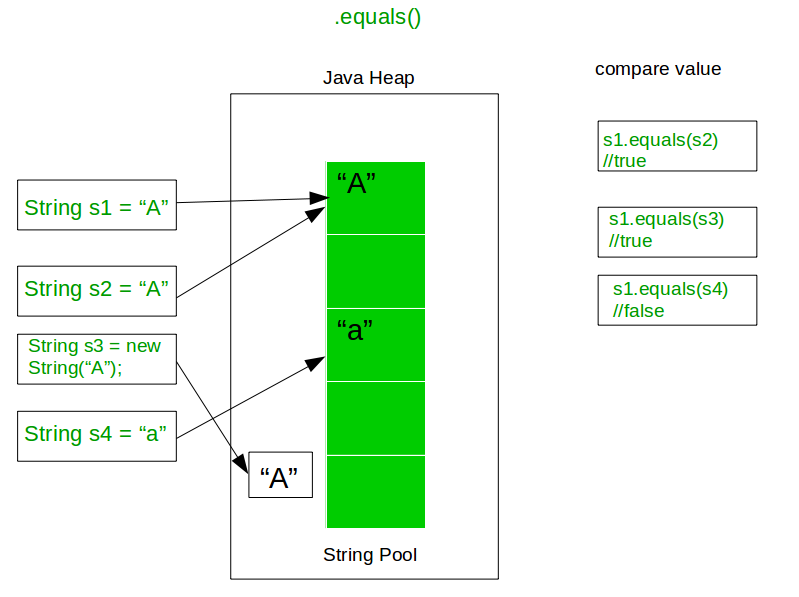# how do I add an if statement to check if firstExample is equal to secondExample?

I am learning Java and I am a bit confused on how I can add an if statement that checks if firstExample is equal to secondExample

Equality.java
```// I have imported a java.io.Console for you, it is named console.
String firstExample = "hello";
String secondExample = "hello";
String thirdExample = "HELLO";
if (secondExample = "hello")) {
console.printf("first is equal to second");
}
```PLUS

You should be aware that the '==' operator compares object references, and not the text itself. So if you later have a string created with the new keyword (another reference) like this:

```String secondExample = new String("hello");
```

and you compare the strings:

```firstExample == secondExample
```

you will get false, e.g. strings are not equal.

So the right way to compare two strings is to use the .equals()-method from the String class itself, like this:

```firstExample.equals(secondExample)
```

this will return true, e.g. strings are equal.

The image below shows how the equals()-method works, and how two strings (s1 and s2) refers to the same object.Use the variable names and == operator in the if statement.

```if(firstExample == secondExample) { // do your stuff }
```